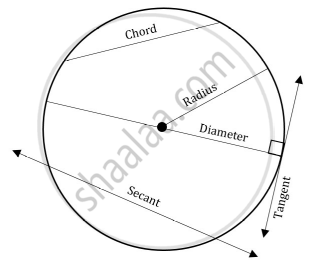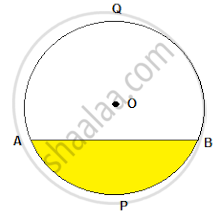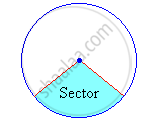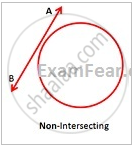Share

# Introduction to Circles

Course

#### definition

A circle is a simple closed shape. It is the set of all points in a plane that are at a given distance from a given point, the centre; equivalently it is the curve traced out by a point that moves in a plane so that its distance from a given point is constant.

#### notes

While learning this chapter we have to be fimiliar with few terms.1) Radius- It is the fixed distance form the centre to the circumference of the circle. Circumference is the boundary of the circle.

2) Diameter- In geometry, a diameter of a circle is any straight line segment that passes through the center of the circle and whose endpoints lie on the circle.

3) Origin- Origin is the centre of the circle. Circle is represented as C (o,r) where o is origin and r is the radius.

4) Chord- A chord of a circle is a straight line segment whose endpoints both lie on the circle. More generally, a chord is a line segment joining two points on any curve. Diameter is the longest chord.

5) Secant- A secant is a line which cuts a circle at two points.

6) Tangent- A straight line that cuts a circle at one single point. The radius of a circle is always perpendicular to the tangent line through its endpoint on the circle's circumference. Conversely, the perpendicular to a radius through the same endpoint is a tangent line, this we will prove in a theorem further.

7) Segment- The segment of a circle is the region bounded by a chord and the arc subtended by the chord.8) Arc-The arc of a circle is a portion of the circumference of a circle. In the figure above AB is a arc in the shaded segment.

9)Sector- The secotor is the portion of a disk enclosed by two radii and an arc, where the smaller area is known as the minor sector and the larger being the major sector.10) Non intersecting line- The line which do not touch the circumference of a circle is known as non intersecting line.### Shaalaa.com

Circles part 1 (Introduction) [00:13:10]
S
0%